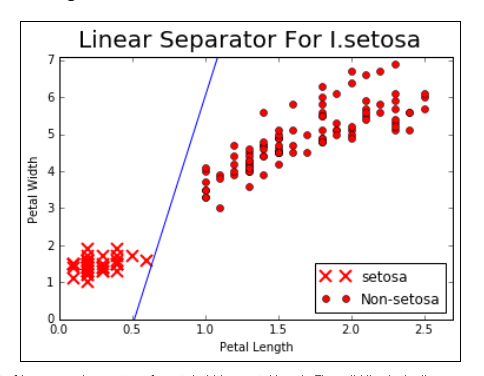# TensorFlow Complete Code

Original article was published by Bhanu Soni on Artificial Intelligence on Medium# TensorFlow Complete Code

## Create a classifier on the iris dataset.

The iris data set is described in more detail in Working with Data Sources in Getting Started with TensorFlow. We will load this data, and do a simple binary classifier to predict whether a flower is a species Iris setosa or not. To be clear, this dataset has three classes of species, but we will only predict whether it is a single species (I. setosa) or not, giving us a binary classifier. We will start by loading the libraries and data, then transform the target accordingly.

Source: TensorFlow Machine Learning Cookbook

## How to do it…

1. First, we load the libraries needed and initialize the computational graph. Note that we also load matplotlib here, because we would like to plot the resulting line after:
`import matplotlib.pyplot as pltimport numpy as npfrom sklearn import datasetsimport tensorflow as tfsess = tf.Session()`

2. Next we load the iris data. We will also need to transform the target data to be just 1 or 0 if the target is setosa or not. Since the iris data set marks setosa as a zero, we will change all targets with the value 0 to 1 , and the other values all to 0. We will also only use two features, petal length and petal width. These two features are the third and fourth entry in each x-value :

`iris = datasets.load_iris()binary_target = np.array([1. if x==0 else 0. for x in iris.target])iris_2d = np.array([[x, x] for x in iris.data])`

3. Let’s declare our batch size, data placeholders, and model variables. Remember that the data placeholders for variable batch sizes have None as the first dimension:

`batch_size = 20x1_data = tf.placeholder(shape=[None, 1], dtype=tf.float32)x2_data = tf.placeholder(shape=[None, 1], dtype=tf.float32)y_target = tf.placeholder(shape=[None, 1], dtype=tf.float32)A = tf.Variable(tf.random_normal(shape=[1, 1]))b = tf.Variable(tf.random_normal(shape=[1, 1]))`

4. Here we define the linear model. The model will take the form of x2=x1*A+b. And if we want to find points above or below that line, we see whether they are above or below zero when plugged into the equation x2-x1*A-b. We will do this by taking the sigmoid of that equation and predicting 1 or 0 from that equation. Remember that TensorFlow has loss functions with the sigmoid built-in, so we just need to define the output of the model prior to the sigmoid function:

`my_mult = tf.matmul(x2_data, A)my_add = tf.add(my_mult, b)my_output = tf.sub(x1_data, my_add)`

5. Now we add our sigmoid cross-entropy loss function with TensorFlow’s built in function, sigmoid_cross_entropy_with_logits() :

`xentropy = tf.nn.sigmoid_cross_entropy_with_logits(my_output, y_target)`

6. We also have to tell TensorFlow how to optimize our computational graph by declaring an optimizing method. We will want to minimize the cross-entropy loss. We will also choose 0.05 as our learning rate:

`my_opt = tf.train.GradientDescentOptimizer(0.05)train_step = my_opt.minimize(xentropy)`

7.Now we create a variable initialization operation and tell TensorFlow to execute it:

`init = tf.initialize_all_variables()sess.run(init)`

8. Now we will train our linear model with 1000 iterations. We will feed in the three data points that we require: petal length, petal width, and the target variable. Every 200 iterations we will print the variable values:

`for i in range(1000):    rand_index = np.random.choice(len(iris_2d), size=batch_size)    rand_x = iris_2d[rand_index]    rand_x1 = np.array([[x] for x in rand_x])    rand_x2 = np.array([[x] for x in rand_x])    rand_y = np.array([[y] for y in binary_target[rand_index]])    sess.run(train_step, feed_dict={x1_data: rand_x1, x2_data:rand_x2, y_target: rand_y})    if (i+1)%200==0:        rint('Step #' + str(i+1) + ' A = ' + str(sess.run(A)) +', b = ' + str(sess.run(b)))Step #200 A = [[ 8.67285347]], b = [[-3.47147632]]Step #400 A = [[ 10.25393486]], b = [[-4.62928772]]Step #600 A = [[ 11.152668]], b = [[-5.4077611]]Step #800 A = [[ 11.81016064]], b = [[-5.96689034]]Step #1000 A = [[ 12.41202831]], b = [[-6.34769201]]`

9. The next set of commands extracts the model variables and plots the line on a graph. The resulting graph is in the next section:

`[[slope]] = sess.run(A)[[intercept]] = sess.run(b)x = np.linspace(0, 3, num=50)ablineValues = []for i in x:    ablineValues.append(slope*i+intercept)    setosa_x = [a for i,a in enumerate(iris_2d) if binary_target[i]==1]    setosa_y = [a for i,a in enumerate(iris_2d) if binary_target[i]==1]    non_setosa_x = [a for i,a in enumerate(iris_2d) if binary_target[i]==0]    non_setosa_y = [a for i,a in enumerate(iris_2d) if binary_    target[i]==0]    plt.plot(setosa_x, setosa_y, 'rx', ms=10, mew=2, label='setosa''')    plt.plot(non_setosa_x, non_setosa_y, 'ro', label='Non-setosa')    plt.plot(x, ablineValues, 'b-')    plt.xlim([0.0, 2.7])    plt.ylim([0.0, 7.1])    plt.suptitle('Linear' Separator For I.setosa', fontsize=20)    plt.xlabel('Petal Length')    plt.ylabel('Petal Width')    plt.legend(loc='lower right')    plt.show()`

## How it works…

Our goal was to fit a line between the I.setosa points and the other two species using only petal width and petal length. If we plot the points and the resulting line, we see that we have achieved the following:

# SUMMARY

we achieved our objective of separating the two classes with a line, it may not be the best model for separating two classes. Support vector machines, which is a better way of separating two classes in a feature space.